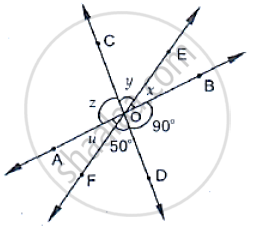# In the Below Fig, Three Coplanar Lines Intersect at a Point O, Forming Angles as Shown in the Figure. Find the Values of X, Y, Z and U. - Mathematics

In the below fig, three coplanar lines intersect at a point O, forming angles as shown in the figure. Find the values of x, y, z and u.#### Solution

Vertically opposite angles are equal

So ∠BOD = z = 90°

∠DOF = y = 50°

Now,x + y + z = 180°                [Linear pair]

⇒ x + y + z = 180°

⇒ 90° + 50° + x = 180°

⇒  x = 180° -140°

⇒  x = 40°

Concept: Concept of Parallel Lines
Is there an error in this question or solution?

#### APPEARS IN

RD Sharma Mathematics for Class 9
Chapter 10 Lines and Angles
Exercise 10.3 | Q 2 | Page 22